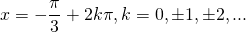# Solution assignment 03 Trigonometric equations

### Assignment 3

Solve the following equations: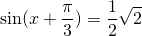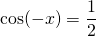### Solution

Looking at the first equation we use the table: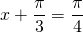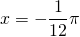but also: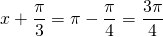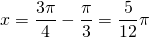Because the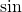-function has period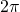, we find the following solution: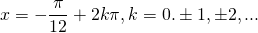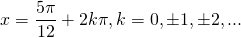For the second equation we have: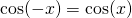and thus the equation is: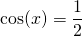and from the table we know that: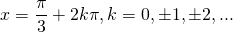or: